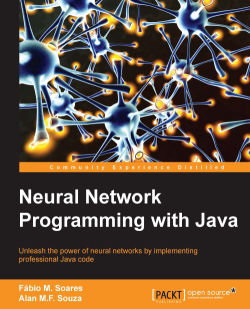### Neural Network Programming with Java4.5 (15 reviews total)
By Alan M. F. Souza , Fabio M. Soares
• FREE Subscribe Access now
• \$44.99 Print + eBook
• \$35.99 eBook
• Constantly updated with 100+ new titles each month
• Breadth and depth in over 1,000+ technologies

Vast quantities of data are produced every second. In this context, neural networks become a powerful technique to extract useful knowledge from large amounts of raw, seemingly unrelated data. One of the most preferred languages for neural network programming is Java as it is easier to write code using it, and most of the most popular neural network packages around already exist for Java. This makes it a versatile programming language for neural networks.

This book gives you a complete walkthrough of the process of developing basic to advanced practical examples based on neural networks with Java.

You will first learn the basics of neural networks and their process of learning. We then focus on what Perceptrons are and their features. Next, you will implement self-organizing maps using the concepts you’ve learned. Furthermore, you will learn about some of the applications that are presented in this book such as weather forecasting, disease diagnosis, customer profiling, and characters recognition (OCR). Finally, you will learn methods to optimize and adapt neural networks in real time.

All the examples generated in the book are provided in the form of illustrative source code, which merges object-oriented programming (OOP) concepts and neural network features to enhance your learning experience.

Publication date:
January 2016
Publisher
Packt
Pages
244
ISBN
9781785880902

## ChapterÂ 1.Â Getting Started with Neural Networks

In this chapter, we will introduce neural networks and what they are designed for. This chapter serves as a foundation layer for the subsequent chapters, while it presents the basic concepts for neural networks. In this chapter, we will cover the following:

• Artificial Neurons

• Weights and Biases

• Activation Functions

• Layers of Neurons

• Neural Network Implementation in Java

## Discovering neural networks

First, the term "neural networks" may create a snapshot of a brain in our minds, particularly for those who have just been introduced to it. In fact, that's right, we consider the brain to be a big and natural neural network. However, what if we talk about artificial neural networks (ANNs)? Well, here comes an opposite word to natural, and the first thing now that comes into our head is an image of an artificial brain or a robot, given the term "artificial." In this case, we also deal with creating a structure similar to and inspired by the human brain; therefore, this can be called artificial intelligence. So, the reader who doesn't have any previous experience with ANN now may be thinking that this book teaches how to build intelligent systems, including an artificial brain, capable of emulating the human mind using Java codes, isn't it? Of course, we will not cover the creation of artificial thinking machines such as those from the Matrix trilogy movies; however, this book will discuss several incredible capabilities that these structures can do. We will provide the reader with Java codes for defining and creating basic neural network structures, taking advantage of the entire Java programming language framework.

## Why artificial neural network?

We cannot begin talking about neural networks without understanding their origins, including the term as well. We use the terms neural networks (NN) and ANN interchangeably in this book, although NNs are more general, covering the natural neural networks as well. So, what actually is an ANN? Let's explore a little of the history of this term.

In the 1940s, the neurophysiologist Warren McCulloch and the mathematician Walter Pits designed the first mathematical implementation of an artificial neuron combining the neuroscience foundations with mathematical operations. At that time, many studies were being carried out on understanding the human brain and how and if it could be simulated, but within the field of neuroscience. The idea of McCulloch and Pits was a real novelty because it added the math component. Further, considering that the brain is composed of billions of neurons, each one interconnected with another million, resulting in some trillions of connections, we are talking about a giant network structure. However, each neuron unit is very simple, acting as a mere processor capable to sum and propagate signals.

On the basis of this fact, McCulloch and Pits designed a simple model for a single neuron, initially to simulate the human vision. The available calculators or computers at that time were very rare but capable of dealing with mathematical operations quite well; on the other hand, even today tasks such as vision and sound recognition are not easily programmed without the use of special frameworks, as opposed to the mathematical operations and functions. Nevertheless, the human brain can perform these latter tasks more efficiently than the first ones, and this fact really instigates scientists and researchers.

So, an ANN is supposed to be a structure to perform tasks such as pattern recognition, learning from data, and forecasting trends, just like an expert can do on the basis of knowledge, as opposed to the conventional algorithmic approach that requires a set of steps to be performed to achieve a defined goal. An ANN instead has the capability to learn how to solve some task by itself, because of its highly interconnected network structure.

Classification of images

Voice recognition

Face identification

Forecast events on the basis of experience

Complex calculation

Grammatical error correction

Signal processing

Operating system management

## How neural networks are arranged

It can be said that the ANN is a nature-inspired structure, so it does have similarities with the human brain. As shown in the following figure, a natural neuron is composed of a nucleus, dendrites, and axon. The axon extends itself into several branches to form synapses with other neurons' dendrites.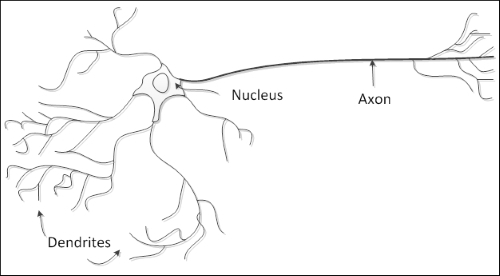So, the artificial neuron has a similar structure. It contains a nucleus (processing unit), several dendrites (analogous to inputs), and one axon (analogous to output), as shown in the following figure: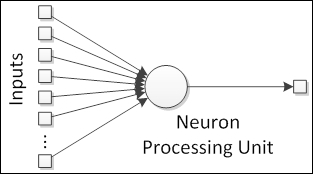The links between neurons form the so-called neural network, analogous to the synapses in the natural structure.

### The very basic element â€“ artificial neuron

Natural neurons have proven to be signal processors since they receive micro signals in the dendrites that can trigger a signal in the axon depending on their strength or magnitude. We can then think of a neuron as having a signal collector in the inputs and an activation unit in the output that can trigger a signal that will be forwarded to other neurons. So, we can define the artificial neuron structure as shown in the following figure: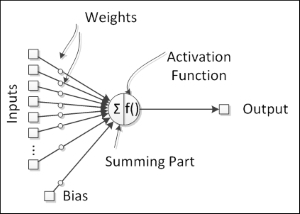### Tip

In natural neurons, there is a threshold potential that when reached, fires the axon and propagates the signal to the other neurons. This firing behavior is emulated with activation functions, which have proven to be useful in representing nonlinear behaviors in the neurons.

### Giving life to neurons â€“ activation function

The neuron's output is given by an activation function. This component adds nonlinearity to neural network processing, which is needed because the natural neuron has nonlinear behaviors. An activation function is usually bounded between two values at the output, therefore being a nonlinear function, but in some special cases, it can be a linear function.

The four most used activation functions are as follows:

• Sigmoid

• Hyperbolic tangent

• Hard limiting threshold

• Purely linear

The equations and charts associated with these functions are shown in the following table:

Function

Equation

Chart

Sigmoid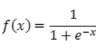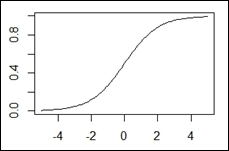Hyperbolic tangent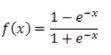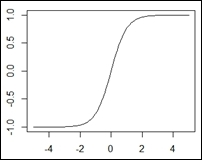Hard limiting threshold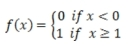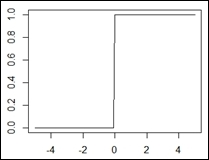Linear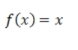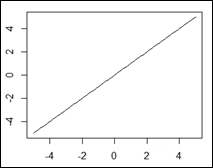### The fundamental values â€“ weights

In neural networks, weights represent the connections between neurons and have the capability to amplify or attenuate neuron signals, for example, multiply the signals, thus modifying them. So, by modifying the neural network signals, neural weights have the power to influence a neuron's output, therefore a neuron's activation will be dependent on the inputs and on the weights. Provided that the inputs come from other neurons or from the external world, the weights are considered to be a neural network's established connections between its neurons. Thus, since the weights are internal to the neural network and influence its outputs, we can consider them as neural network knowledge, provided that changing the weights will change the neural network's capabilities and therefore actions.

### An important parameter â€“ bias

The artificial neuron can have an independent component that adds an extra signal to the activation function. This component is called bias.

Just like the inputs, biases also have an associated weight. This feature helps in the neural network knowledge representation as a more purely nonlinear system.

### The parts forming the whole â€“ layers

Natural neurons are organized in layers, each one providing a specific level of processing; for example, the input layer receives direct stimuli from the outside world, and the output layers fire actions that will have a direct influence on the outside world. Between these layers, there are a number of hidden layers, in the sense that they do not interact directly with the outside world. In the artificial neural networks, all neurons in a layer share the same inputs and activation function, as shown in the following figure: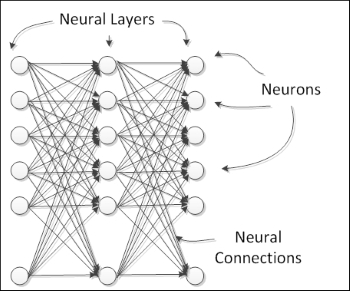Neural networks can be composed of several linked layers, forming the so-called multilayer networks. The neural layers can be basically divided into three classes:

• Input layer

• Hidden layer

• Output layer

In practice, an additional neural layer adds another level of abstraction of the outside stimuli, thereby enhancing the neural network's capacity to represent more complex knowledge.

### Tip

Every neural network has at least an input/output layer irrespective of the number of layers. In the case of a multilayer network, the layers between the input and the output are called hidden.

## Learning about neural network architectures

Basically, a neural network can have different layouts, depending on how the neurons or neuron layers are connected to each other. Every neural network architecture is designed for a specific end. Neural networks can be applied to a number of problems, and depending on the nature of the problem, the neural network should be designed in order to address this problem more efficiently.

Basically, there are two modalities of architectures for neural networks:

• Neuron connections

• Monolayer networks

• Multilayer networks

• Signal flow

• Feedforward networks

• Feedback networks

### Monolayer networks

In this architecture, all neurons are laid out in the same level, forming one single layer, as shown in the following figure:The neural network receives the input signals and feeds them into the neurons, which in turn produce the output signals. The neurons can be highly connected to each other with or without recurrence. Examples of these architectures are the single-layer perceptron, Adaline, self-organizing map, Elman, and Hopfield neural networks.

### Multilayer networks

In this category, neurons are divided into multiple layers, each layer corresponding to a parallel layout of neurons that shares the same input data, as shown in the following figure: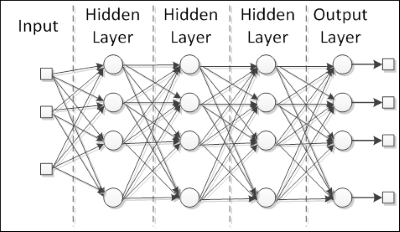Radial basis functions and multilayer perceptrons are good examples of this architecture. Such networks are really useful for approximating real data to a function specially designed to represent that data. Moreover, because they have multiple layers of processing, these networks are adapted to learn from nonlinear data, being able to separate it or determine more easily the knowledge that reproduces or recognizes this data.

### Feedforward networks

The flow of the signals in neural networks can be either in only one direction or in recurrence. In the first case, we call the neural network architecture feedforward, since the input signals are fed into the input layer; then, after being processed, they are forwarded to the next layer, just as shown in the figure in the multilayer section. Multilayer perceptrons and radial basis functions are also good examples of feedforward networks.

### Feedback networks

When the neural network has some kind of internal recurrence, it means that the signals are fed back in a neuron or layer that has already received and processed that signal, the network is of the type feedback. See the following figure of feedback networks: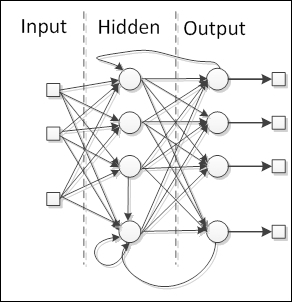The special reason to add recurrence in the network is the production of a dynamic behavior, particularly when the network addresses problems involving time series or pattern recognition, that require an internal memory to reinforce the learning process. However, such networks are particularly difficult to train, eventually failing to learn. Most of the feedback networks are single layer, such as Elman and Hopfield networks, but it is possible to build a recurrent multilayer network, such as echo and recurrent multilayer perceptron networks.

## From ignorance to knowledge â€“ learning process

Neural networks learn by adjusting the connections between the neurons, namely the weights. As mentioned in the neural structure section, weights represent the neural network knowledge. Different weights cause the network to produce different results for the same inputs. So, a neural network can improve its results by adapting its weights according to a learning rule. The general schema of learning is depicted in the following figure: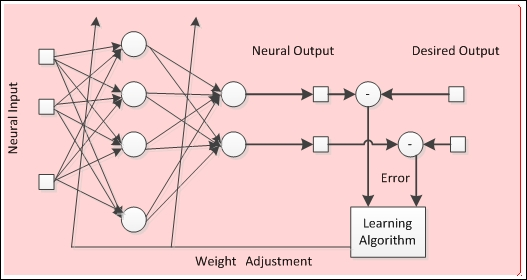The process depicted in the preceding figure is called supervised learning because there is a desired output, but neural networks can learn only by the input data, without any desired output (supervision). In Chapter 2, How Neural Networks Learn, we are going to dive deeper into the neural network learning process.

## Let the implementations begin! Neural networks in practice

In this book, we will cover the entire process of implementing a neural network by using the Java programming language. Java is an object-oriented programming language that was created in the 1990s by a small group of engineers from Sun Microsystems, later acquired by Oracle in the 2010s. Nowadays, Java is present in many devices that are part of our daily life.

In an object-oriented language, such as Java, we deal with classes and objects. A class is a blueprint of something in the real world, and an object is an instance of this blueprint, something like a car (class referring to all and any car) and my car (object referring to a specific carâ€”mine). Java classes are usually composed of attributes and methods (or functions), that include objects-oriented programming (OOP) concepts. We are going to briefly review all of these concepts without diving deeper into them, since the goal of this book is just to design and create neural networks from a practical point of view. Four concepts are relevant and need to be considered in this process:

• Abstraction: The transcription of a real-world problem or rule into a computer programming domain, considering only its relevant features and dismissing the details that often hinder development.

• Encapsulation: Analogous to a product encapsulation by which some relevant features are disclosed openly (public methods), while others are kept hidden within their domain (private or protected), therefore avoiding misuse or excess of information.

• Inheritance: In the real world, multiple classes of objects share attributes and methods in a hierarchical manner; for example, a vehicle can be a superclass for car and truck. So, in OOP, this concept allows one class to inherit all features from another one, thereby avoiding the rewriting of code.

• Polymorphism: Almost the same as inheritance, but with the difference that methods with the same signature present different behaviors on different classes.

Using the neural network concepts presented in this chapter and the OOP concepts, we are now going to design the very first class set that implements a neural network. As can be seen, a neural network consists of layers, neurons, weights, activation functions, and biases, and there are basically three types of layers: input, hidden, and output. Each layer may have one or more neurons. Each neuron is connected either to a neural input/output or to another neuron, and these connections are known as weights.

It is important to highlight that a neural network may have many hidden layers or none, as the number of neurons in each layer may vary. However, the input and output layers have the same number of neurons as the number of neural inputs/outputs, respectively.

So, let's start implementing. Initially, we are going to define six classes, detailed as follows:

 Class name: Neuron Attributes `private ArrayList listOfWeightIn` An `ArrayList` variable of real numbers that represents the list of input weights `private ArrayList listOfWeightOut` An `ArrayList` variable of real numbers that represents the list of output weights Methods `public double initNeuron()` Initializes `listOfWeightIn` and `listOfWeightOut` function with a pseudo random real number Parameters: None Returns: A pseudo random real number `public void setListOfWeightIn(ArrayList listOfWeightIn)` Sets the `listOfWeightIn` function with a list of real numbers list Parameters: The list of real numbers to be stored in the class object Returns: None `public void setListOfWeightOut(ArrayList listOfWeightOut)` Sets the `listOfWeightOut` function with a list of real numbers list Parameters: The list of real numbers to be stored in the class object Returns: None `public ArrayList getListOfWeightIn()` Returns the input weights a list of neurons Parameters: None Returns: The list of real numbers stored in the `listOfWeightIn` variable `public ArrayList getListOfWeightOut()` Returns the output weights a list of neurons Parameters: None Returns: The list of real numbers stored in the `listOfWeightOut` variable Class implementation with Java: file Neuron.java Class Name: Layer Note: This class is abstract and cannot be instantiated. Attributes `private ArrayList listOfNeurons` An `ArrayList` variable of objects of the Neuron class `private int numberOfNeuronsInLayer` Integer number to store the quantity of neurons that are part of the layer Methods `public ArrayList getListOfNeurons()` Returns the list of neurons by layer Parameters: None Returns: An `ArrayList` variable of objects by the `Neuron` class `public void setListOfNeurons(ArrayList listOfNeurons)` Sets the `listOfNeurons` function with an `ArrayList` variable of objects of the `Neuron` class Parameters: The list of objects of the `Neuron` class to be stored Returns: None `public int getNumberOfNeuronsInLayer()` Returns the number of neurons by layer Parameters: None Returns: The number of neurons by layer `public void setNumberOfNeuronsInLayer(int numberOfNeuronsInLayer)` Sets the number of neurons in a layer Parameters: The number of neurons in a layer Returns: None Class implementation with Java: file Layer.java Class name: InputLayer Note: This class inherits attributes and methods from the Layer class. Attributes None Methods `public initLayer(InputLayer inputLayer)` Initializes the input layer with pseudo random real numbers Parameters: An object of the `InputLayer `class Returns: None `public void printLayer(InputLayer inputLayer)` Prints the input weights of the layer Parameters: An object of the `InputLayer `class Returns: None Class implementation with Java: file InputLayer.java Class name: HiddenLayer Note: This class inherits attributes and methods from the Layer class. Attributes None Methods `public ArrayList initLayer(HiddenLayer hiddenLayer, ArrayList listOfHiddenLayer, InputLayer inputLayer, OutputLayer outputLayer)` Initializes the hidden layer(s) with pseudo random real numbers Parameters: An object of the `HiddenLayer `class, a list of objects of the `HiddenLayer `class, an object of the `InputLayer `class, an object of the `OutputLayer `class Returns: None `public void printLayer(ArrayList listOfHiddenLayer)` Prints the weights of the layer(s) Parameters: A list of objects of the `HiddenLayer `class Returns: None Class implementation with Java: file HiddenLayer.java Class name: OutputLayer Note: This class inherits attributes and methods from the Layer class. Attributes None Methods `public OutputLayer initLayer(OutputLayer outputLayer)` Initializes the output layer with pseudo random real numbers Parameters: An object of the `OutputLayer `class Returns: None `public void printLayer(OutputLayer outputLayer)` Prints the weights of the layer Parameters: An object of the `OutputLayer `class Returns: None Class implementation with Java: file OutputLayer.java Class name: NeuralNet Note: The values of the neural net topology are fixed in this class (two neurons in the input layer, two hidden layers with three neurons each, and one neuron in the output layer). Reminder: It's the first version. Attributes `private InputLayer inputLayer;` An object of the `InputLayer `class `private HiddenLayer hiddenLayer;` An object of the `HiddenLayer `class `private ArrayList listOfHiddenLayer;` An `ArrayList` variable of objects of the `HiddenLayer `class. It is possible to have more than one hidden layer `private OutputLayer outputLayer;` An object of the `OutputLayer` class `private int numberOfHiddenLayers;` Integer number to store the quantity of layers that are part of the hidden layer Methods `public void initNet()` Initializes the neural net as a whole. Layers are built, and each list of the weights of neurons is built randomly Parameters: None Returns: None `public void printNet()` Prints the neural net as a whole. Each input and output weight of each layer is shown Parameters: None Returns: None Class implementation with Java: file NeuralNet.java

One advantage of OOP languages is the ease to document the program in Unified Modeling Language (UML). UML class diagrams present classes, attributes, methods, and relationships between classes in a very simple and straightforward manner, thus helping the programmer and/or stakeholders to understand the project as a whole. The following figure represents the very first version of the project's class diagram: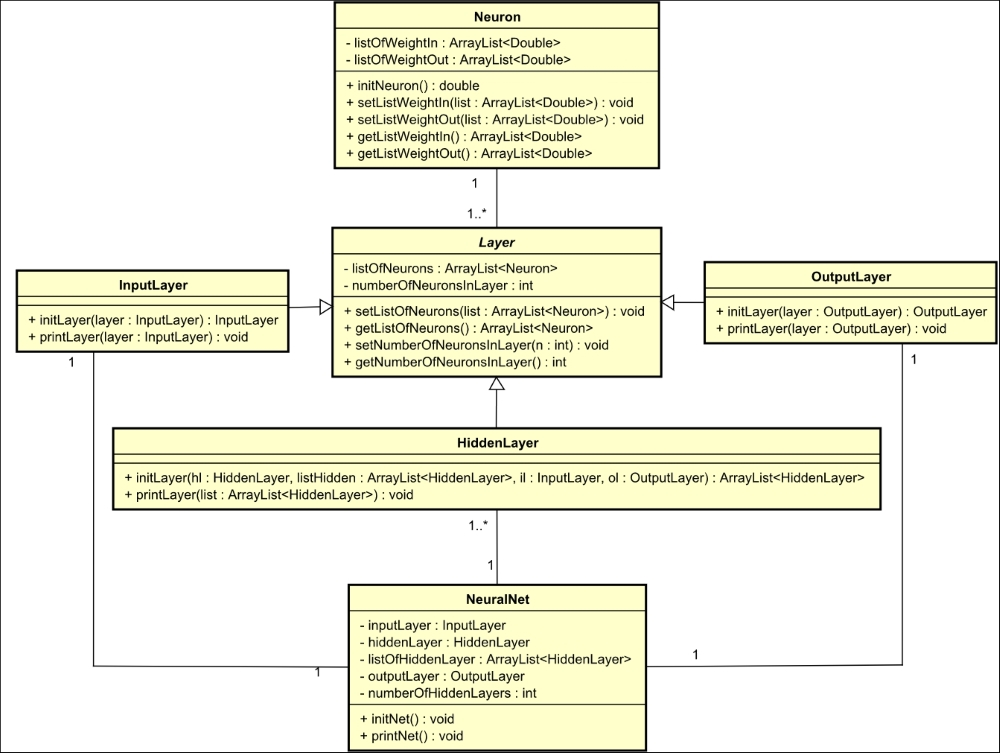Now, let's apply these classes and get some results. The code shown next has a test class, a main method with an object of the `NeuralNet` class called `n`. When this method is called (by executing the class), it calls the `initNet()` and `printNet()`methods from the object `n`, generating the following result shown in the figure right after the code. It represents a neural network with two neurons in the input layer, three in the hidden layer, and one in the output layer:

```public class NeuralNetTest {
public static void main(String[] args) {
NeuralNet n = new NeuralNet();
n.initNet();
n.printNet();

}
}```

It's relevant to remember that each time that the code runs, it generates new pseudo random weight values. So, when you run the code, the other values will appear in Console: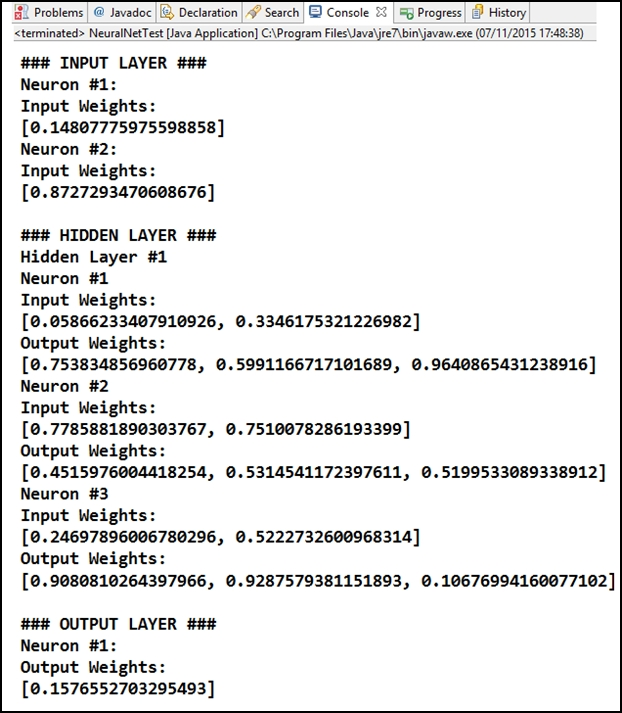## Summary

In this chapter, we've seen an introduction to the neural networks, what they are, what they are used for, and their basic concepts. We've also seen a very basic implementation of a neural network in the Java programming language, wherein we applied the theoretical neural network concepts in practice, by coding each of the neural network elements. It's important to understand the basic concepts before we move on to advanced concepts. The same applies to the code implemented with Java.

In the next chapter, we will delve into the learning process of a neural network and explore the different types of leaning with simple examples.

• ##### Alan M. F. Souza

Alan M. F. Souza is computer engineer from Instituto de Estudos Superiores da Amazonia (IESAM). He holds a post-graduate degree in project management software and a master's degree in industrial processes (applied computing) from Universidade Federal do Para (UFPA). He has been working with neural networks since 2009 and has worked with Brazilian IT companies developing in Java, PHP, SQL, and other programming languages since 2006. He is passionate about programming and computational intelligence. Currently, he is a professor at Universidade da Amazonia (UNAMA) and a PhD candidate at UFPA.

Browse publications by this author
• ##### Fabio M. Soares

Fábio M. Soares is currently a PhD candidate at the Federal University of Pará (Universidade Federal do Pará - UFPA), in northern Brazil. He is very passionate about technology in almost all fields, and designs neural network solutions since 2004 and has applied this technique in several fields like telecommunications, industrial process control and modeling, hydroelectric power generation, financial applications, retail customer analysis and so on. His research topics cover supervised learning for data-driven modeling. As of 2017, he is currently carrying on research projects with chemical process modeling and control in the aluminum smelting and ferronickel processing industries, and has worked as a lecturer teaching subjects involving computer programming and artificial intelligence paradigms. As an active researcher, he has also a number of articles published in English language in many conferences and journals, including four book chapters.

Browse publications by this author

### Latest Reviews

(15 reviews total)